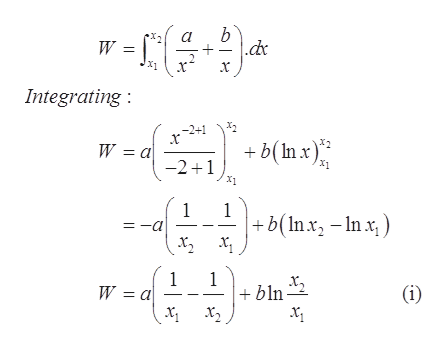# Consider an object of mass m = 4 kg on a frictionless table. The object experiences a repulsive force F = a/x2 + b/x, where F is in newtons and x is the position of the object relative to the origin.Write an expression for the work done by the repulsive force on the object as it moves from an initial position of x1 to a final position of x2.  If the object starts at a position of x1 = 8.5 m apart, how much work, in joules, is required by an external force to bring it to a position of x2 = 2.5 m apart when a = 19 and b = 11?  If the object starts at rest at a position x3 = 1.3 m and is released, at what speed v, in meters per second, will the object be moving when it is at position x4 = 12.5 m?

Question
58 views

Consider an object of mass m = 4 kg on a frictionless table. The object experiences a repulsive force F = a/x2 + b/x, where F is in newtons and x is the position of the object relative to the origin.

Write an expression for the work done by the repulsive force on the object as it moves from an initial position of x1 to a final position of x2

If the object starts at a position of x1 = 8.5 m apart, how much work, in joules, is required by an external force to bring it to a position of x2 = 2.5 m apart when a = 19 and b = 11?

If the object starts at rest at a position x3 = 1.3 m and is released, at what speed v, in meters per second, will the object be moving when it is at position x4 = 12.5 m?

check_circle

Step 1

Given:

Mass of the object, m = 4 kg

Repulsive force, F = a/x2 + b/x

Step 2

The formula of work done:

Step 3

Calculation for the expression of work done:

Pluggin...help_outlineImage Transcriptioncloseb .dc W = x Integrating -2+1 b(In x) W — а -21 X1 1 =-a 1 +b(Inx-Inx х, х, 1 W 3 а 1 bln х, х,, (i) fullscreen

### Want to see the full answer?

See Solution

#### Want to see this answer and more?

Solutions are written by subject experts who are available 24/7. Questions are typically answered within 1 hour.*

See Solution
*Response times may vary by subject and question.
Tagged in

### Other